#### NCERT Solutions for Class 8th: Ch 3 Understanding Quadrilaterals Maths Part-II

Page No: 50

Exercise 3.3

1. Given a parallelogram ABCD. Complete each statement along with the definition or property used.DCB = ......
(iii) OC = ......         (iv) m ∠DAB + m ∠CDA = ......

(i) AD = BC   (Opposite sides of a parallelogram are equal)
(ii) ∠DCB = ∠DAB    (Opposite angles of a parallelogram are equal)
(iii) OC = OA    (Diagonals of a parallelogram are equal)
(iv)  m ∠DAB + m ∠CDA = 180°

Page No: 51

2. Consider the following parallelograms. Find the values of the unknowns x, y, z.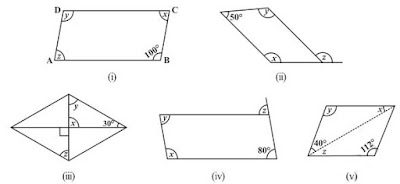(i)y = 100°   (opposite angles of a parallelogram)
x + 100° = 180°   (Adjacent angles of a parallelogram)
⇒ x = 180° - 100° = 80°
x = z = 80°   (opposite angles of a parallelogram)
Thus, x = 80°, y = 100° and y = 100°

(ii)50° + x = 180° ⇒ x = 180° - 50° = 130° (Adjacent angles of a parallelogram)

x = y = 130°   (opposite angles of a parallelogram)
x = z = 130°   (corresponding angle)

(iii)x = 90°   (vertical opposite angles)
x + y + 30° = 180°   (angle sum property of a triangle)
⇒ 90° + y + 30° = 180°
⇒ y = 180° - 120° = 60°
also, y = z = 60°   (alternate angles)

(iv)z = 80°   (corresponding angle)
z = y = 80°   (alternate angles)
x + y = 180°   (adjacent angles)
⇒ x + 80° = 180° ⇒ x = 180° - 80° = 100°

3. Can a quadrilateral ABCD be a parallelogram if
(i) ∠D + ∠B = 180°?         (ii) AB = DC = 8 cm, AD = 4 cm and BC = 4.4 cm?
(iii) ∠A = 70° and ∠C = 65°?

(i)Yes, a quadrilateral ABCD be a parallelogram if ∠D + ∠B = 180° but it should also fulfilled some conditions which are:
• The sum of the adjacent angles should be 180°.
• Opposite angles must be equal.

(ii) No, opposite sides should be of same length. Here, AD ≠ BC

(iii) No, opposite angles should be of same measures. ∠A ≠ ∠C

4. Draw a rough figure of a quadrilateral that is not a parallelogram but has exactly two opposite angles of equal measure.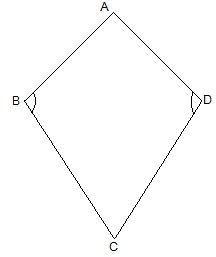ABCD is a figure of quadrilateral that is not a parallelogram but has exactly two opposite angles that is ∠B = ∠D of equal measure. It is not a parallelogram because ∠A ≠ ∠C.

5. The measures of two adjacent angles of a parallelogram are in the ratio 3 : 2. Find the measure of each of the angles of the parallelogram.

Let the measures of two adjacent angles ∠A and ∠B be 3x and 2x respectively in parallelogram ABCD.
∠A + ∠B = 180°
⇒ 3x + 2x = 180°
⇒ 5x = 180°
⇒ x = 36°
We know that opposite sides of a parallelogram are equal.
∠A = ∠C = 3x = 3 × 36° = 108°
∠B = ∠D = 2x = 2 × 36° = 72°

6. Two adjacent angles of a parallelogram have equal measure. Find the measure of each of the angles of the parallelogram.

Let ABCD be a parallelogram.
Sum of adjacent angles of a parallelogram =  180°
∠A + ∠B = 180°
⇒ 2∠A = 180°
⇒ ∠A = 90°
also, 90° + ∠B = 180°
⇒ ∠B = 180° - 90° = 90°
∠A = ∠C = 90°
∠B = ∠D = 90°

7. The adjacent figure HOPE is a parallelogram. Find the angle measures x, y and z. State the properties you use to find them.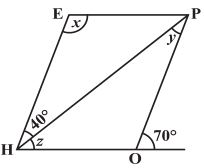y = 40°   (alternate interior angle)
∠P = 70°   (alternate interior angle)
∠P = ∠H = 70°   (opposite angles of a parallelogram)
z = ∠H - 40°= 70° - 40° = 30°
∠H + x = 180°
⇒ 70° + x = 180°
⇒ x = 180° - 70° = 110°

8. The following figures GUNS and RUNS are parallelograms. Find x and y. (Lengths are in cm)(i) SG = NU and SN = GU   (opposite sides of a parallelogram are equal)
3x = 18
⇒ x = 18/3 = 6
3y - 1 = 26 and,
⇒ 3y = 26 + 1
⇒ y = 27/3 = 9
x = 6 and y = 9

(ii) 20 = y + 7 and 16 = x + y   (diagonals of a parallelogram bisect each other)
y + 7 = 20
⇒ y = 20 - 7 = 13 and,
x + y  = 16
⇒ x + 13 = 16
⇒ x = 16 - 13 = 3
x = 3 and y = 13

9. In the above figure both RISK and CLUE are parallelograms. Find the value of x.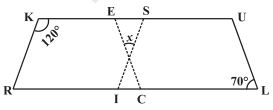∠K + ∠R = 180°   (adjacent angles of a parallelogram are supplementary)
⇒ 120° + ∠R = 180°
⇒ ∠R = 180° - 120° = 60°
also, ∠R = ∠SIL   (corresponding angles)
⇒ ∠SIL = 60°
also, ∠ECR = ∠L = 70°   (corresponding angles)
x + 60° + 70° = 180°   (angle sum of a triangle)
⇒ x + 130° = 180°
⇒ x = 180° - 130° = 50°

Page No: 52

10. Explain how this figure is a trapezium. Which of its two sides are parallel? (Fig 3.32)When a transversal line intersects two lines in such a way that the sum of the adjacent angles on the same side of transversal is 180° then the lines are parallel to each other.
Here, ∠M + ∠L = 100° + 80° = 180°
Thus, MN || LK
As the quadrilateral KLMN has one pair of parallel line therefore it is a trapezium.
MN and LK are parallel lines.

11. Find m∠C in Fig 3.33 if  AB || DC ?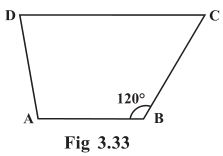m∠C + m∠B = 180°   (angles on the same side of transversal)
⇒ m∠C + 60° = 180°
⇒ m∠C = 180°- 60° = 120°

12. Find the measure of ∠P and ∠S if  SP || RQ ? in Fig 3.34. (If you find m∠R, is there more than one method to find m∠P?)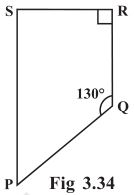∠P + ∠Q = 180°   (angles on the same side of transversal)
⇒ ∠P + 130° = 180°
⇒ ∠P = 180° - 130° = 50°
also, ∠R + ∠S = 180°   (angles on the same side of transversal)
⇒ 90° + ∠S = 180°
⇒ ∠S = 180° - 90° = 90°
Thus, ∠P = 50° and ∠S = 90°

Yes, there are more than one method to find m∠P.
PQRS is a quadrilateral. Sum of measures of all angles is 360°.
Since, we know the measurement of ∠Q, ∠R and ∠S.
∠Q = 130°, ∠R = 90° and ∠S = 90°
∠P + 130° + 90° + 90° = 360°
⇒ ∠P + 310° = 360°
⇒ ∠P = 360° - 310° = 50°

Page No: 55

Exercise 3.4

1. State whether True or False.
(a) All rectangles are squares.
(b) All rhombuses are parallelograms.
(c) All squares are rhombuses and also rectangles.
(d) All squares are not parallelograms.
(e) All kites are rhombuses.
(f) All rhombuses are kites.
(g) All parallelograms are trapeziums.
(h) All squares are trapeziums.

(a) False. All square are rectangles but all rectangles are not square.
(b) True
(c) True
(d) False. All squares are parallelograms as opposite sides are parallel and opposite angles are equal.
(e) False. A kite doesn't have all sides of same length.
(f) True
(g) True
(h) True

2. Identify all the quadrilaterals that have.
(a) four sides of equal length       (b) four right angles

(a) Rhombus and square have all four sides of equal length.
(b) Square and rectangle have four right angles.

3. Explain how a square is.
(i) a quadrilateral    (ii) a parallelogram   (iii) a rhombus    (iv) a rectangle

(i) Square is a quadrilateral because it has four sides.
(ii) Square is a parallelogram because its opposite sides are parallel and opposite angles are equal.
(iii) Square is a rhombus because its all four sides of equal length and diagonals bisect at right angles.
(iv) Square is a rectangle because its each interior angle is 90°

4. Name the quadrilaterals whose diagonals.
(i) bisect each other    (ii) are perpendicular bisectors of each other    (iii) are equal

(i) Parallelogram, Rhombus, Square and Rectangle
(ii) Rhombus and Square
(iii) Rectangle and Square

5. Explain why a rectangle is a convex quadrilateral.

Rectangle is a convex quadrilateral because its both diagonals lie inside the rectangle.

6. ABC is a right-angled triangle and O is the mid point of the side opposite to the right angle. Explain why O is equidistant from A, B and C. (The dotted lines are drawn additionally to help you).